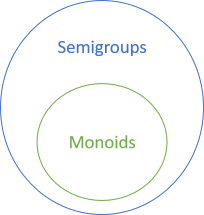Introduction to semigroups for object-oriented programmers.Semigroups form a superset of monoids. They are associative binary operations. While monoids additionally require that an identity element exists, no such requirement exist for semigroups. In other words, all monoids are semigroups, but not all semigroups are monoids.

This article gives you an overview of semigroups, as well as a few small examples. A supplemental article will show a more elaborate example.

### Minimum #

An operation that returns the smallest of two values form a semigroup. In the .NET Base Class Library, such an operation is already defined for many numbers, for example 32-bit integers. Since associativity is a property of a semigroup, it makes sense to demonstrate it with a property-based test, here using FsCheck:

```[Property(QuietOnSuccess = true)]
public void IntMinimumIsAssociative(int x, int y, int z)
{
Assert.Equal(
Math.Min(Math.Min(x, y), z),
Math.Min(x, Math.Min(y, z)));
}```

This example uses the FsCheck.Xunit glue library for xUnit.net. Notice that although FsCheck is written in F#, you can also use it from C#. This test (as well as all other tests in this article) passes.

For mathematical integers, no identity element exists, so the minimum operation doesn't form a monoid. In practice, however, .NET 32-bit integers do have an identity element:

```[Property(QuietOnSuccess = true)]
public void MimimumIntHasIdentity(int x)
{
Assert.Equal(x, Math.Min(int.MaxValue, x));
Assert.Equal(x, Math.Min(x, int.MaxValue));
}```

Int32.MaxValue is the maximum possible 32-bit integer value, so it effectively behaves as the identity for the 32-bit integer minimum operation. All 32-bit numbers are smaller than, or equal to, `Int32.MaxValue`. This effectively makes `Math.Min(int, int)` a monoid, but conceptually, it's not.

This may be clearer if, instead of 32-bit integers, you consider BigInteger, which is an arbitrarily large (or small) integer. The minimum operation is still associative:

```[Property(QuietOnSuccess = true)]
public void BigIntMinimumIsAssociative(
BigInteger x,
BigInteger y,
BigInteger z)
{
Assert.Equal(
BigInteger.Min(BigInteger.Min(x, y), z),
BigInteger.Min(x, BigInteger.Min(y, z)));
}```

No identity element exists, however, because no matter which integer you have, you can always find one that's bigger: no maximum value exists. This makes `BigInteger.Min` a semigroup, but not a monoid.

### Maximum #

Like minimum, the maximum operation forms a semigroup, here demonstrated by `BigInteger.Max`:

```[Property(QuietOnSuccess = true)]
public void BigIntMaximumIsAssociative(
BigInteger x,
BigInteger y,
BigInteger z)
{
Assert.Equal(
BigInteger.Max(BigInteger.Max(x, y), z),
BigInteger.Max(x, BigInteger.Max(y, z)));
}```

Again, like minimum, no identity element exists because the set of integers is infinite; you can always find a bigger or smaller number.

Minimum and maximum operations aren't limited to primitive numbers. If values can be compared, you can always find the smallest or largest of two values, here demonstrated with `DateTime` values:

```[Property(QuietOnSuccess = true)]
public void DateTimeMaximumIsAssociative(
DateTime x,
DateTime y,
DateTime z)
{
Func<DateTime, DateTime, DateTime> dtMax =
(dt1, dt2) => dt1 > dt2 ? dt1 : dt2;
Assert.Equal(
dtMax(dtMax(x, y), z),
dtMax(x, dtMax(y, z)));
}```

As was the case with 32-bit integers, however, the presence of DateTime.MinValue effectively makes `dtMax` a monoid, but conceptually, no identity element exists, because dates are infinite.

### First #

Another binary operation simply returns the first of two values:

```public static T First<T>(T x, T y)
{
return x;
}```

This may seem pointless, but `First` is associative:

```[Property(QuietOnSuccess = true)]
public void FirstIsAssociative(Guid x, Guid y, Guid z)
{
Assert.Equal(
First(First(x, y), z),
First(x, First(y, z)));
}```

On the other hand, there's no identity element, because there's no left identity. The left identity is an element `e` such that `First(e, x) == x` for any `x`. Clearly, for any generic type `T`, no such element exists because `First(e, x)` will only return `x` when `x` is equal to `e`. (There are, however, degenerate types for which an identity exists for `First`. Can you find an example?)

### Last #

Like `First`, a binary operation that returns the last (second) of two values also forms a semigroup:

```public static T Last<T>(T x, T y)
{
return y;
}```

Similar to `First`, `Last` is associative:

```[Property(QuietOnSuccess = true)]
public void LastIsAssociative(String x, String y, String z)
{
Assert.Equal(
Last(Last(x, y), z),
Last(x, Last(y, z)));
}```

As is also the case for `First`, no identity exists for `Last`, but here the problem is the lack of a right identity. The right identity is an element `e` for which `Last(x, e) == x` for all `x`. Clearly, `Last(x, e)` can only be equal to `x` if `e` is equal to `x`.

### Aggregation #

Perhaps you think that operations like `First` and `Last` seem useless in practice, but when you have a semigroup, you can reduce any non-empty sequence to a single value. In C#, you can use the Aggregate LINQ method for this. For example

```var a = new[] { 1, 0, 1337, -10, 42 }.Aggregate(Math.Min);
```

returns `-10`, while

```var a = new[] { 1, 0, 1337, -10, 42 }.Aggregate(Math.Max);
```

returns `1337`. Notice that the input sequence is the same in both examples, but the semigroup differs. Likewise, you can use `Aggregate` with `First`:

```var a = new[] { 1, 0, 1337, -10, 42 }.Aggregate(First);
```

Here, `a` is `1`, while

```var a = new[] { 1, 0, 1337, -10, 42 }.Aggregate(Last);
```

returns `42`.

LINQ has specialised methods like Min, Last, and so on, but from the perspective of behaviour, `Aggregate` would have been enough. While there may be performance reasons why some of those specialised methods exist, you can think of all of them as being based on the same abstraction: that of a semigroup.

`Aggregate`, and many of the specialised methods, throw an exception if the input sequence is empty. This is because there's no identity element in a semigroup. The method doesn't know how to create a value of the type `T` from an empty list.

If, on the other hand, you have a monoid, you can return the identity element in case of an empty sequence. Haskell explicitly makes this distinction with `sconcat` and `mconcat`, but I'm not going to go into that now.

### Summary #

Semigroups are associative binary operations. In the previous article series about monoids you saw plenty of examples, and since all monoids are semigroups, you've already seen more than one semigroup example. In this article, however, you saw four examples of semigroups that are not monoids.

All four examples in this article are simple, and may not seem like 'real-world' examples. In the next article, then, you'll get a more realistic example of a semigroup that's not a monoid.

Next: Bounding box semigroup.

### Wish to comment?

You can add a comment to this post by sending me a pull request. Alternatively, you can discuss this post on Twitter or somewhere else with a permalink. Ping me with the link, and I may respond.

#### Published

Monday, 27 November 2017 12:39:00 UTC

#### Tags

"Our team wholeheartedly endorses Mark. His expert service provides tremendous value."
Hire me!
Published: Monday, 27 November 2017 12:39:00 UTC